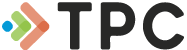• Online Training• Instructor-Led Training• Performance• Compliance• TECHNOLOGY• Industries• Resources• Contact
• ## Foundations of Technology# Force and Motion

The online Force and Motion course covers fundamentals of force and motion, showing how an engineer thinks about these concepts. Demonstrates how mathematical and graphical representations can help clarify our thinking about mechanical force and motion. This course has no prerequisites. Force and Motion is available in online maintenance training and course manual formats.

TPC Training is authorized by IACET to offer 0.8 CEUs for this program.

Review a full course list for Mechanical Systems Technician

Back to Foundations of Technology Courses

#### Select Format

• Online Course & Test \$85.00
• Course Manual \$66.00

TPC Training is authorized by IACET to offer 0.8 CEUs for Force and Motion online training.

#### Lesson 1 - Scalars and Vectors

##### Topics:

Physical quantities; Properties and components of vectors; Adding, subtracting, multiplying, and dividing vectors

##### Learning Objectives:
– Explain the difference between scalars and vectors, and list examples of each.
– Draw a vector, given a verbal description.
– Describe a vector verbally, given its graphic symbol, a frame of reference, and an appropriate scale.
– Define resolution, resultant, and commutative.
– Demonstrate how to resolve a vector into its rectangular components.
– Demonstrate how to add and subtract vector quantities in one-dimensional and two-dimensional frames of reference.
– Multiply and divide a vector by a scalar.

#### Lesson 2 - Motion Along a Straight Line

##### Topics:

Speed; Velocity; Slope; Changing and instantaneous velocity; Velocity-vs-time graph; Finding displacement; Using a curved graph

##### Learning Objectives:
– Explain the difference between speed and velocity.
– Define the terms instantaneous velocity, average velocity, and slope.
– Identify the delta notation, and explain how to use it in a calculation.
– Demonstrate how to determine displacement and velocity from a position-versus-time graph.
– Demonstrate how to determine displacement by calculating the area under a velocity-versus-time graph.

#### Lesson 3 - Acceleration

##### Topics:

Directional acceleration; Equations of motion; Acceleration due to gravity; Upward and lateral motion during free fall

##### Learning Objectives:
– Define acceleration.
– Demonstrate how to determine the magnitude of an acceleration from a velocity-verses-time graph.
– Demonstrate the difference between average acceleration and instantaneous acceleration.
– Solve simple problems for average acceleration, displacement, and final velocity.
– Explain why the velocity-verses-time graph for all objects in free fall are parallel (when drawn on the same coordinate system).

#### Lesson 4 - How to Describe Force

##### Topics:

Motion; Basic forces of nature; Action/reaction pairs; Newton's Third Law of Motion; Unbalanced forces; Resolution of forces

##### Learning Objectives:
– Define force.
– Name the four basic types of forces in nature.
– State Newton’s Universal Law of Gravitation.
– Explain how and why vectors are used to represent forces.
– Explain Newton’s Third Law of Motion.
– Give examples which demonstrate that forces always occur in action-reaction pairs.
– Demonstrate how to add force vectors.
– Describe how to resolve a force vector into its components.

#### Lesson 5 - Force and Acceleration

##### Topics:

Friction; Newton's First and Second Laws of Motion; "Resistance" to acceleration; Units of force; Conservation of momentum

##### Learning Objectives:
– State Newton’s First Law of Motion.
– Define inertia, and describe how it is measured.
– Explain why a force must be applied continuously to objects on earth in order to maintain their motion.
– Explain what happens when a net applied force is greater than the friction force.
– State Newton’s Second Law of Motion.
– Solve problems using the equation F=ma.

#### Lesson 6 - Equilibrium

##### Topics:

Forces on bodies; Particles in static equilibrium; Equilibrium of a rigid body; Center of mass

##### Learning Objectives:
– State the two conditions of equilibrium, and distinguish between static and dynamic equilibrium.
– Explain the difference between particles and rigid bodies.
– Define torque.
– Solve problems involving torque and rotational equilibrium.

#### Lesson 7 - Rotational and Circular Motion

##### Topics:

Centripetal and centrifugal force; Rotational motion; Angular displacement; Velocity; Acceleration; Tangential acceleration; Moments of inertia

##### Learning Objectives:
– Describe centripetal force.
– Differentiate between centripetal acceleration, angular acceleration, and tangential acceleration, and state the formula for each.
– Demonstrate how to convert degree measurements to radian measurements.
– Define the terms uniform circular motion, period angular velocity, angular impulse, angular momentum, and moment of inertia.
– Explain why moment of inertia is calculated differently for different objects.
– State Newton;s Second Law in terms of rotational motion.
– Identify examples of the Law of Conservation of Angular Momentum.

#### Lesson 8 - Simple Harmonic Motion

##### Topics:

Periodic motion; Simple harmonic motion; Hooke's Law; Equations of harmony; Resonance

##### Learning Objectives:
– Describe the relationship between simple harmonic motion and uniform circular motion.
– Define the terms cycle, amplitude, frequency, period, and Hertz.
– State Hooke’s Law.
– Describe how acceleration and restoring force vectors change as an object moves in simple harmonic motion.
– Use equations to determine the period and frequency of both a mass-spring system and a pendulum.
– Give examples of resonance.